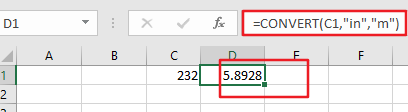# How to Convert the Unit of Measurement for Cells in Excel

This post will guide you how to convert the unit of measurement from one unit to another in Excel. How do I convert various unit measurements in cells with a formula in Excel 2013/2016.

## Convert Unit of Measurement

Assuming that you have a list of data that contain numeric values, and you want to convert a measurement from one unit to anther, such as: from “inch” to “meter”. You can use the CONVERT function to convert the unit of measurement for cells. You just need to enter data and units into the following formula:

=CONVERT(number,”from_unit”,”to_unit”)

For example, you want to change the unit of measurement for cell C1 from inch to meter, you can use the following formula:

`=CONVERT(C1,”in”,”m”)`

Note: you need to enter in the approved abbreviated form of the original unit into the second parameter, such as: “in”, and then enter in the approved abbreviated form for “to_unit” in the third parameter.

You need enter this formula into a blank cell and press Enter key on your keyboard to apply this formula.Then you would see that the unit measurement has been converted.

Related Posts

Calculate Interest for Given Period

Using the IPMT function in Excel, we can compute the interest payment on any loan. This step-by-step tutorial will guide Excel users of all skill levels through the process to calculate interest for given period. Finally, the formula: =IPMT(B3/12,1,B5,-B2) The ...

How To Use Excel GCD Function

This post will guide you how to use Excel GCD function with syntax and examples in Microsoft excel. Description The Excel GCD function Returns the greatest common divisor of two or more integers. So you can use the GCD function ...

Calculate A Ratio From Two Numbers In Excel

In elementary mathematics, a ratio is a connection or comparison between two or more integers. For example, ratios are often expressed as ":" to demonstrate the relationship between two numbers. You would think that manually calculating a ratio from two ...

How To Use Excel RRI Function

This post will guide you how to use Excel RRI function with syntax and examples in Microsoft excel. Description The Excel RRI function Returns an equivalent interest rate for the growth of an investment. So you can use the RRI ...

CAGR Formula Examples in Excel

CAGR in Excel is a formula that calculates the compound annual growth rate for any invested amount over the specified years or timeframe. Although there is no direct function in Excel that can help us identify the CAGR value, there ...

Build Hyperlink With VLOOKUP in Excel

You might have come across a task in which you were assigned to build hyperlinks, which seems very easy, and if you are new to excel or don't have enough experience with it, then you might wonder about doing this ...

Break ties with helper COUNTIF and column

Suppose you got a task to adjust the values that contain the ties; what would be your first attempt to break the ties of the given value? If you are wondering about doing this task manually, let me add that ...

Calculate Total Cost with Excel VLOOKUP Function

In today's article we will show you how to calculate the total cost for a given weight using the Excel VLOOKUP function. This function will help us to find the appropriate unit price for that weight and then we can ...

Excel Formulas To Calculate The Bond Valuation

Assume that you've been assigned a task of calculating bond valuation; so if you are new to Ms Excel or do not have much experience with it, then I am pretty sure about it that doing this task manually might ...

Calculate Cumulative Totals with Excel SUM Function

Today, through a simple example, we will show you how to use one of the most common-used Mathematical functions in excel, the SUM function, to add up the sum. In our daily life, we keep an account of what we ...

Sidebar